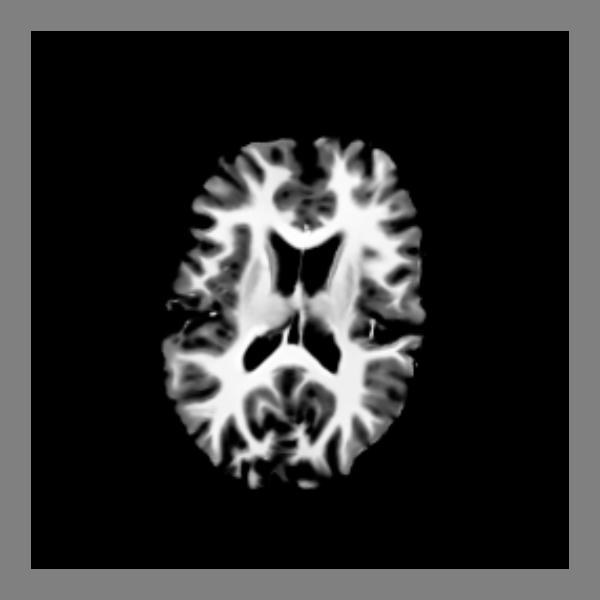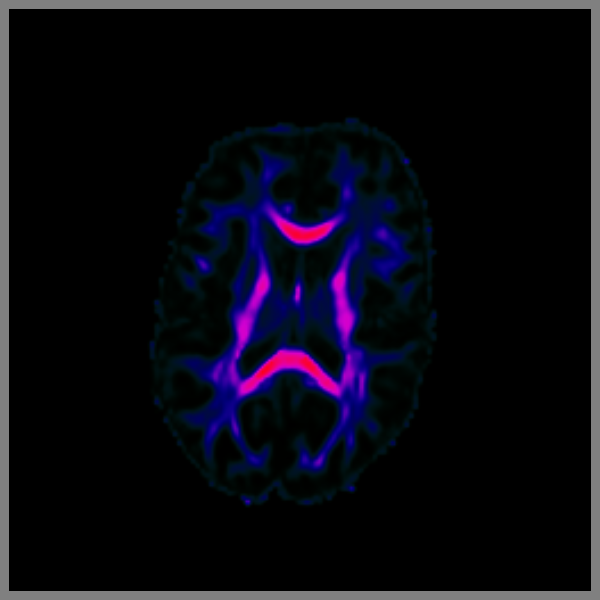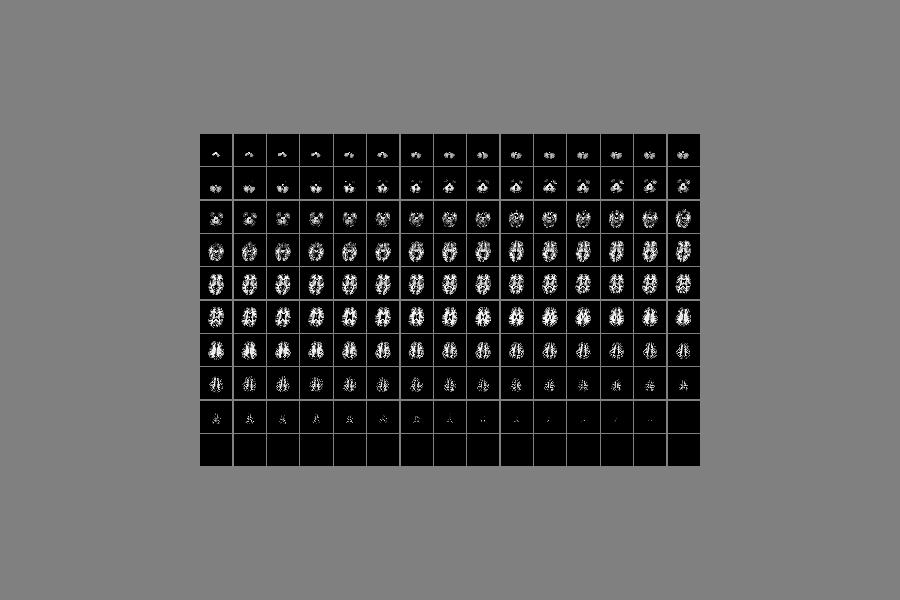# Simple volume slicing¶

Here we present an example for visualizing slices from 3D images.

```import os
import nibabel as nib
from dipy.data import fetch_bundles_2_subjects
from fury import window, actor, ui
```

```fetch_bundles_2_subjects()

fname_t1 = os.path.join(os.path.expanduser('~'), '.dipy',
'exp_bundles_and_maps', 'bundles_2_subjects',
'subj_1', 't1_warped.nii.gz')

data = img.get_data()
affine = img.affine
```

Out:

```Data size is approximately 234MB
Dataset is already in place. If you want to fetch it again please first remove the folder /Users/koudoro/.dipy/exp_bundles_and_maps
```

Create a Scene object which holds all the actors which we want to visualize.

```scene = window.Scene()
scene.background((0.5, 0.5, 0.5))
```

## Render slices from T1 with a specific value range¶

The T1 has usually a higher range of values than what can be visualized in an image. We can set the range that we would like to see.

```mean, std = data[data > 0].mean(), data[data > 0].std()
value_range = (mean - 0.5 * std, mean + 1.5 * std)
```

The `slice` function will read data and resample the data using an affine transformation matrix. The default behavior of this function is to show the middle slice of the last dimension of the resampled data.

```slice_actor = actor.slicer(data, affine, value_range)
```

The `slice_actor` contains an axial slice.

```scene.add(slice_actor)
```

The same actor can show any different slice from the given data using its `display` function. However, if we want to show multiple slices we need to copy the actor first.

```slice_actor2 = slice_actor.copy()
```

Now we have a new `slice_actor` which displays the middle slice of sagittal plane.

```slice_actor2.display(slice_actor2.shape//2, None, None)

scene.reset_camera()
scene.zoom(1.4)
```

In order to interact with the data you will need to uncomment the line below.

```# window.show(scene, size=(600, 600), reset_camera=False)
```

Otherwise, you can save a screenshot using the following command.

```window.record(scene, out_path='slices.png', size=(600, 600),
reset_camera=False)
```## Render slices from FA with your colormap¶

```# It is also possible to set the colormap of your preference. Here we are
# loading an FA image and showing it in a non-standard way using an HSV
# colormap.

fname_fa = os.path.join(os.path.expanduser('~'), '.dipy',
'exp_bundles_and_maps', 'bundles_2_subjects',
'subj_1', 'fa_1x1x1.nii.gz')

fa = img.get_data()
```

Notice here how the scale range is. We use FA min and max values to set it up

```lut = actor.colormap_lookup_table(scale_range=(fa.min(), fa.max()),
hue_range=(0.4, 1.),
saturation_range=(1, 1.),
value_range=(0., 1.))
```

This is because the lookup table is applied in the slice after interpolating to (0, 255).

```fa_actor = actor.slicer(fa, affine, lookup_colormap=lut)

scene.clear()

scene.reset_camera()
scene.zoom(1.4)

# window.show(scene, size=(600, 600), reset_camera=False)

window.record(scene, out_path='slices_lut.png', size=(600, 600),
reset_camera=False)
```Now we would like to add the ability to click on a voxel and show its value on a panel in the window. The panel is a UI element which requires access to different areas of the visualization pipeline and therefore we don’t recommend using it with `window.show`. The more appropriate way is to use the `ShowManager` object, which allows accessing the pipeline in different areas.

```show_m = window.ShowManager(scene, size=(1200, 900))
show_m.initialize()
```

We’ll start by creating the panel and adding it to the `ShowManager`

```label_position = ui.TextBlock2D(text='Position:')
label_value = ui.TextBlock2D(text='Value:')

result_position = ui.TextBlock2D(text='')
result_value = ui.TextBlock2D(text='')

panel_picking = ui.Panel2D(size=(250, 125),
position=(20, 20),
color=(0, 0, 0),
opacity=0.75,
align="left")

```

Add a left-click callback to the slicer. Also disable interpolation so you can see what you are picking.

```def left_click_callback(obj, _ev):
"""Get the value of the clicked voxel and show it in the panel."""
event_pos = show_m.iren.GetEventPosition()

obj.picker.Pick(event_pos,
event_pos,
0,
show_m.scene)

i, j, k = obj.picker.GetPointIJK()
result_position.message = '({}, {}, {})'.format(str(i), str(j), str(k))
result_value.message = '%.8f' % data[i, j, k]

fa_actor.SetInterpolate(False)

# show_m.start()
```

## Create a mosaic¶

By using the `copy` and `display` method of the `slice_actor` becomes easy and efficient to create a mosaic of all the slices.

So, let’s clear the scene and change the projection from perspective to parallel. We’ll also need a new show manager and an associated callback.

```scene.clear()
scene.projection('parallel')

result_position.message = ''
result_value.message = ''

show_m_mosaic = window.ShowManager(scene, size=(1200, 900))
show_m_mosaic.initialize()

def left_click_callback_mosaic(obj, _ev):
"""Get the value of the clicked voxel and show it in the panel."""
event_pos = show_m_mosaic.iren.GetEventPosition()

obj.picker.Pick(event_pos,
event_pos,
0,
show_m_mosaic.scene)

i, j, k = obj.picker.GetPointIJK()
result_position.message = '({}, {}, {})'.format(str(i), str(j), str(k))
result_value.message = '%.8f' % data[i, j, k]
```

Now we need to create two nested for loops which will set the positions of the grid of the mosaic and add the new actors to the scene. We are going to use 15 columns and 10 rows but you can adjust those with your datasets.

```cnt = 0

X, Y, Z = slice_actor.shape[:3]

rows = 10
cols = 15
border = 10

for j in range(rows):
for i in range(cols):
slice_mosaic = slice_actor.copy()
slice_mosaic.display(None, None, cnt)
slice_mosaic.SetPosition((X + border) * i,
0.5 * cols * (Y + border) - (Y + border) * j,
0)
slice_mosaic.SetInterpolate(False)
left_click_callback_mosaic,
1.0)
cnt += 1
if cnt > Z:
break
if cnt > Z:
break

scene.reset_camera()
scene.zoom(1.0)

```window.record(scene, out_path='mosaic.png', size=(900, 600),